This post introduces Neural Networks, which are ideally suited to extracting features from images. We will use them to classify images of digits (0-9) from the MNIST dataset, which is a mix of digits written by high school students and employees of the United States Census Bureau.

In this post, you'll learn how to:

• train and evaluate a Neural Network.

By the end, you'll learn how to predict the following digits: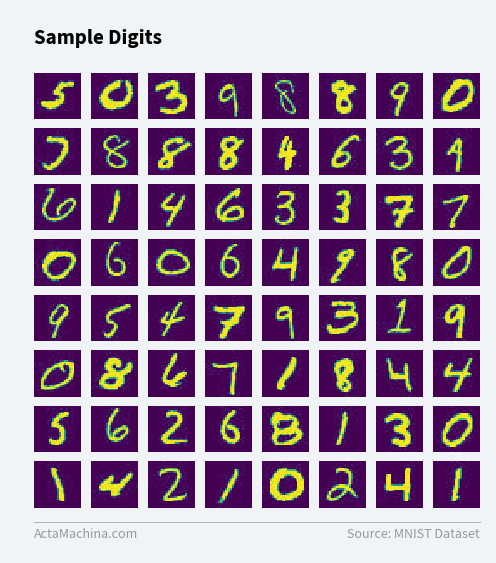## Import Packages¶

We'll be using the PyTorch package from Facebook to build and train the Neural Networks.

In :
from torchvision import datasets, transforms
from torchvision.utils import make_grid

from torch.utils.data.dataset import random_split

import torch
import torch.nn as nn
import torch.nn.functional as F
import torch.optim as optim


## Neural Networks¶

### Architecture¶

Here is a Neural Network that can be used to classify the digits from the MNIST dataset:

Out: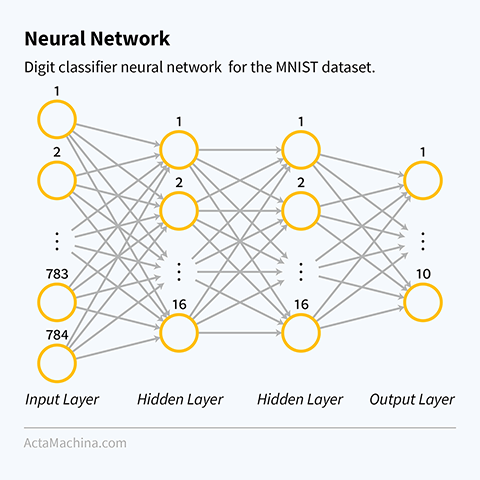This Neural Network has 3 types of layers:

• input layer: each digit image (28 x 28 pixels) is flattened into a vector of size 784 (since 28 x 28 = 784) and fed into the Neural Network as its inputs. The value of each input neuron is a number between 0 and 1 that represents the intensity of that pixel, with 0 being black and 1 being the white.
• hidden layer: each neuron in the hidden layer (meaning not the input or output layers) takes in a number of inputs from the previous layer, each with an associated weight. The neuron then combines them and forwards the output to the neurons in the next layer that it's connected to.
• output layer: since this is a classification task, each output neuron in the output layer corresponds to a class. Based on the cost function we use, the output neuron with the largest value is the predicted class, e.g. if neuron 8 has the largest value, then the network predicts the image to contain the digit 8.

A Neural Network always consists of one input layer, one output layer and one or more hidden layers.

Out: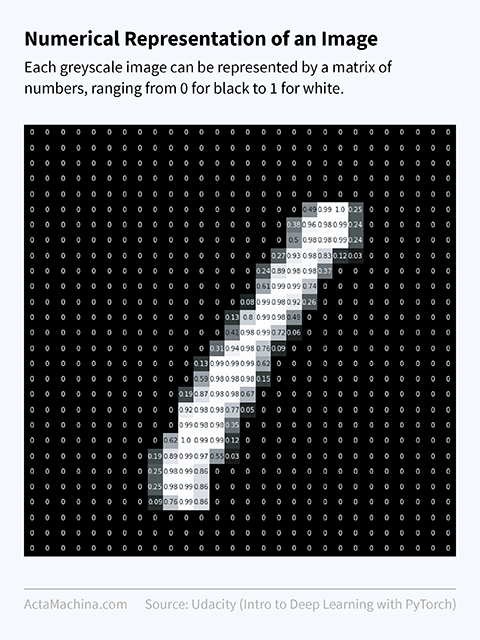### Forward Pass¶

The forward pass of a Neural Network is the process of traversing the Neural Network from input to output, one layer at a time, in order to calculate the output. It is needed in both the training and evaluation (or prediction) phases:

• training phase: for each training example, which is an input/output pair, we need the forward pass to calculate the output layer so it can be compared with the ground truth (i.e. the correct answer) in order for adjustments to the Neural Network to be made.
• evaluation phase: we need the forward pass to calculate the output layer, which is then taken as the prediction.

The evaluation phase is sometimes called the testing phase in certain textbooks.

Out: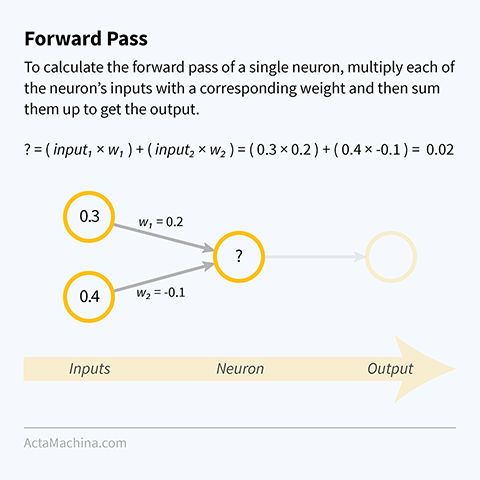### Backpropagation¶

Backpropagation is the process of traversing a Neural Network from output to input, one layer at a time, in order to update the weights of a Neural Network in the training phase.

Out: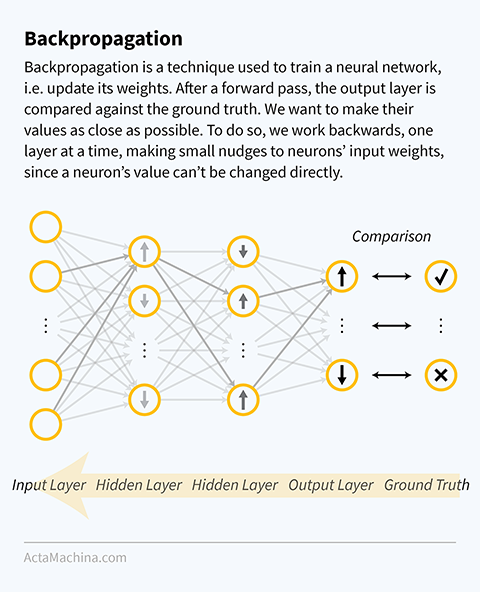### Training the Neural Network¶

To train a Neural Network:

1. Initialise weights of all links between neurons to a random number so that each neuron will act slightly differently.
1. For each pass of the training dataset (called an epoch):

3. For each batch of training examples (input/output pairs) in the training dataset:

4. For each training example (input/output pair) in the batch:

5. Use the input to make a forward pass and get a predicted output.

6. Compare the predicted output with the ground truth output.

7. Use backpropagation to nudge the weights of the neural network in the right direction, bringing the predicted and ground truth outputs closer together.

### Evaluating the Neural Network¶

Evaluation of a Neural Network is another name for using the Neural Network to make predictions. The steps to do this are:

1. For each batch of inputs in the test dataset:

2. For each input in the batch:

3. Use the input to make a forward pass and get a predicted output.

### Alternate Explanation¶

I strongly suggest you take a look at this 1-hour introduction to understanding Neural Networks, consisting of 4 videos by Grant Sanderson: 3Blue1Brown on Neural Networks.

## Implementing Neural Networks with PyTorch¶

Here is the digit classifier Neural Network in the previous section defined in PyTorch:

In :
class Net(nn.Module):
def __init__(self):
super(Net, self).__init__()
self.fc1 = nn.Linear(784, 16, bias=False)
self.fc2 = nn.Linear(16, 16, bias=False)
self.fc3 = nn.Linear(16, 10, bias=False)

def forward(self, x):
x = x.view(-1, 784)  # flatten 28x28 image
x = self.fc1(x)
x = self.fc2(x)
x = self.fc3(x)
return x

net = Net()


PyTorch creates a Neural Network by first initialising all the layers in the __init__ method before using them in the forward method. Our network architecture contains 3 fully-connected layers: from 784 neurons to 16 neurons, 16 neurons to 16 neurons and finally 16 neurons to 10 neurons. In the forward pass, we flatten the 28 x 28 pixel image before passing it to the input layer.

PyTorch provides a host of utility functions that make working with image datasets a lot easier.

In :
transform = transforms.Compose([
transforms.Grayscale(),
transforms.ToTensor(),
])

dataset = datasets.ImageFolder('data/mnist', transform=transform)

train_dataset, test_dataset = random_split(dataset, [len(dataset)-10000, 10000])



Here, we have utilised:

• transforms to pre-process the images into greyscale and tensors;
• ImageFolder to load the images using the directory structure to indicate classes, e.g. for a dataset with two classes, cat and dog, and 4 images:
• data/cat/1.png
• data/cat/2.png
• data/cat/3.png
• ...
• data/dog/1.png
• data/dog/2.png
• data/dog/3.png
• ...
• random_split to randomly split up the dataset into train and test datasets; and
• DataLoader to split the train and test datasets into batches of 64 images and provide an iterator to loop through the batches.

PyTorch also has a number of datasets that can be downloaded within PyTorch including:

• CIFAR10: this dataset contains colour, 32 x 32 pixel images, distributed among 10 classes such as airplane, automobiles, birds, cats, deers, dogs, etc.
• MNIST: a mix of digits written by high school students and employees of the United States Census Bureau. (The dataset we are using in this post.)
• Fashion MNIST: a drop-in replacement for MNIST, this dataset contains greyscale, 28 x 28 pixel images, distributed among 10 classes which are fashion items such as trousers, pullovers, dresses, coats, sandals, etc.

In the training phase, the forward pass calculates the output layer, which is compared to the ground truth in order for backpropagation to know how to nudge the weights. This comparison between the calculated output layer and the ground truth is handled by the criterion function.

In this case, we use CrossEntropyLoss which compares the value of each output neuron with all the others. The resulting difference, or loss, can be expressed mathematically as:

$-\log \left( \frac{\exp\left(\textrm{current output node}\right)}{\exp\left(\textrm{first output node}\right) + \ldots + \exp\left(\textrm{last output node}\right)}\right)$.

The optimizer specificies how the backpropagation algorithm adjusts the weights.

In :
criterion = nn.CrossEntropyLoss()
optimizer = optim.SGD(net.parameters(), lr=0.001, momentum=0.9)


This is the code for the training phase:

In :
net.train()  # set network to training phase

epochs = 2

# for each pass of the training dataset
for epoch in range(epochs):
train_loss, train_correct, train_total = 0, 0, 0

# for each batch of training examples
for batch_index, (inputs, labels) in enumerate(train_dataloader):
outputs = net(inputs)  # forward pass
loss = criterion(outputs, labels)  # compare output with ground truth
loss.backward()  # backpropagation
optimizer.step()  # update network weights

# record statistics
_, preds = torch.max(outputs.data, 1)
train_loss += loss.item()
train_correct += (preds == labels).sum().item()
train_total += len(labels)

# print statistics every 100 batches
if (batch_index + 1) % 100 == 0:
print(f'Epoch {epoch + 1}, ' +
f'Batch {batch_index + 1}, ' +
f'Train Loss: {(train_loss/100):.5f}, ' +
f'Train Accuracy: {(train_correct/train_total):.5f}')

train_loss, train_correct, train_total = 0, 0, 0

Epoch 1, Batch 100, 100 Batch Loss: 2.25285
Epoch 1, Batch 200, 100 Batch Loss: 2.10451
Epoch 1, Batch 300, 100 Batch Loss: 1.87838
Epoch 1, Batch 400, 100 Batch Loss: 1.61642
Epoch 1, Batch 500, 100 Batch Loss: 1.33379
Epoch 1, Batch 600, 100 Batch Loss: 1.10638
Epoch 1, Batch 700, 100 Batch Loss: 0.94047
Epoch 1, Batch 800, 100 Batch Loss: 0.83456
Epoch 1, Batch 900, 100 Batch Loss: 0.74161
Epoch 2, Batch 100, 100 Batch Loss: 0.70251
Epoch 2, Batch 200, 100 Batch Loss: 0.66195
Epoch 2, Batch 300, 100 Batch Loss: 0.61577
Epoch 2, Batch 400, 100 Batch Loss: 0.59637
Epoch 2, Batch 500, 100 Batch Loss: 0.56264
Epoch 2, Batch 600, 100 Batch Loss: 0.53324
Epoch 2, Batch 700, 100 Batch Loss: 0.50598
Epoch 2, Batch 800, 100 Batch Loss: 0.49742
Epoch 2, Batch 900, 100 Batch Loss: 0.46630


Stepping through the code, we see that setting the number of epochs to 2 means that we run through the whole image dataset twice. For each time that we run through the whole dataset, we loop through the batches one-by-one: (i) using the forward pass to make predictions; (ii) using the criterion to calculate a loss; and (iii) backpropagating that loss to work out the gradients at each neuron. The optimiser then takes a step in trying to minimise the loss and updates the weights.

This is the code for the evaluation phase:

In :
net.eval()  # set network to evaluation phase

test_loss = 0
test_correct = 0

# for each batch of testing examples
for batch_index, (inputs, labels) in enumerate(test_dataloader, 1):
outputs = net(inputs)  # forward pass

# record loss
loss = criterion(outputs, labels)
test_loss += loss.item()

# select largest output as prediction
_, preds = torch.max(outputs.data, 1)

# compare prediction with ground truth and mark as correct if equal
test_correct += (preds == labels).sum().item()

f'Test Accuracy: {(test_correct/test_total):.5f} ' +
f'({test_correct}/{test_total})')

Accuracy: 0.86610 (8661/10000)


In the evaluation phase, we set net.eval() to turn off any settings used in training (we will see more of this in the next post). We also use the context of torch.no_grad() to deactivate tracking of the gradients, which speeds up the running of the network. Then, we loop through all the batches in test_dataloader and predict the most likely case for each image, incrementing the correct counter if the predicted class and the ground truth label are identical.

We see that in this case, the trained network has an accuracy of 86.610% against the test set! We'll be using different techniques to try and improve this in the next post. Let's conclude this post by predicting the sample digits we saw in the Introduction, which are the first batch from test_dataloader.

In :
test_dataloader_iterator = iter(test_dataloader)

# forward pass
outputs = net(inputs)

# select largest output as prediction
_, preds = torch.max(outputs.data, 1)

# compare prediction with ground truth and mark as correct if equal
test_correct = (preds == labels).sum().item()
test_total = len(inputs)

print(np.matrix(preds.view(8, 8)))
print(f'Accuracy: {(test_correct/test_total):.5f} ' +
f'({test_correct}/{test_total})')

[[8 0 3 9 8 8 9 0]
[3 8 8 8 9 5 3 9]
[2 1 9 6 3 3 7 7]
[0 6 0 6 4 7 8 0]
[9 5 4 7 9 3 1 9]
[0 5 6 7 1 8 4 4]
[5 6 2 6 2 1 3 0]
[1 4 2 1 0 2 4 1]]
Accuracy: 0.84375 (54/64)


# Bonus: Faster Neural Networks using GPUs¶

Computer graphics have long involved matrix calculations and transformations, which as it turns out, is exactly what is needed for training Neural Networks. The Graphics Processing Unit (GPU) on graphics cards are heavily optimised for these types of calculations. This will often result in a 5 x speed increase for training larger models when compared to training on the Central Processing Unit (CPU).

To check whether you have a GPU and how many are accessible by PyTorch, run:

In :
print(f'Available GPUs: {torch.cuda.device_count()}')

Available GPUs: 2


You can set a device global variable to automatically toggle between CPU and GPU:

In :
device = torch.device('cuda:0' if torch.cuda.is_available() else 'cpu')


Now, moving any variables to device will automatically copy them to the GPU if one is present; otherwise it will keep them on the CPU. To use the GPU, the network will need to be moved, as well as any inputs and labels. So, the two relevant lines to add are:

net = net.to(device),

when instantiating the network; and

inputs, labels = inputs.to(device), labels.to(device),

As this is a small network, the speedup is neglible since the network spends more time moving tensors in and out of the GPU than running the actual calculations. The author trained the above network in 17.3s (using the CPU) vs 20.2s (using the GPU).

## Summary and Next Steps¶

In this post, you've learnt how to: